Function Repository Resource:

# LaplaceBeltramiOperator

Compute the Laplace–Beltrami operator of a mesh region

Contributed by: Gabriel St-Pierre-Lemieux (Université d'Ottawa and the National Research Council Canada)
 ResourceFunction["LaplaceBeltramiOperator"][mr] generates the eigenvalues and eigenvectors of the Laplace-Beltrami operator for the mesh region mr.

## Details

ResourceFunction["LaplaceBeltramiOperator"] takes the same options as Eigensystem.
If mr is a BoundaryMeshRegion, LaplaceBeltrami first applies TriangulateMesh to it.
The matrices generated by ResourceFunction["LaplaceBeltramiOperator"] can be really large and the solution can take a lot of time to compute. In most applications, only the few first eigenvalues and eigenvectors are useful.

## Examples

### Basic Examples (4)

A mesh representing the Space Shuttle:

 In:=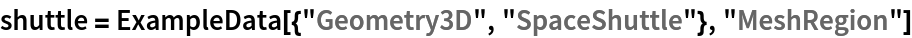Out=Compute the eigensystem of the Laplace-Beltrami operator:

 In:=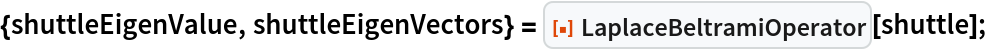Plot the eigenvalues:

 In:=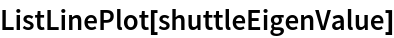Out=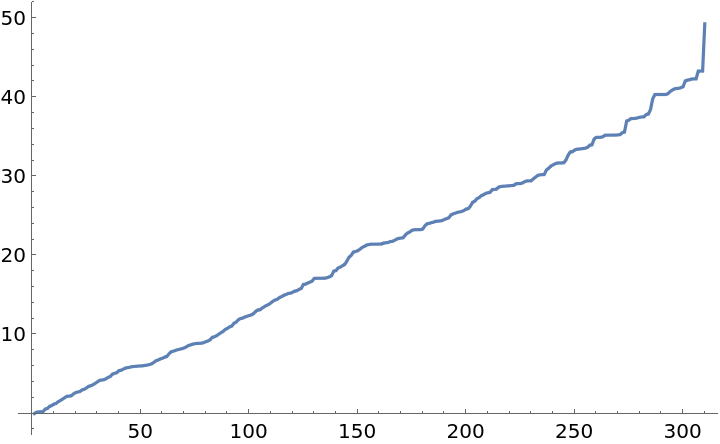Visualize the eigenvectors on the mesh region:

 In:=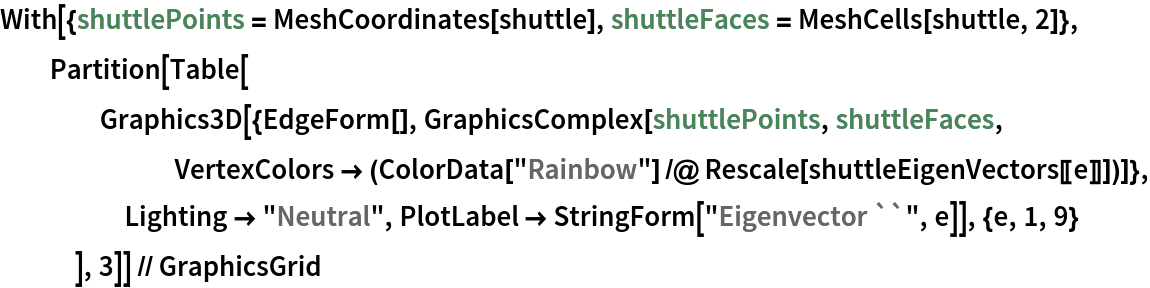Out=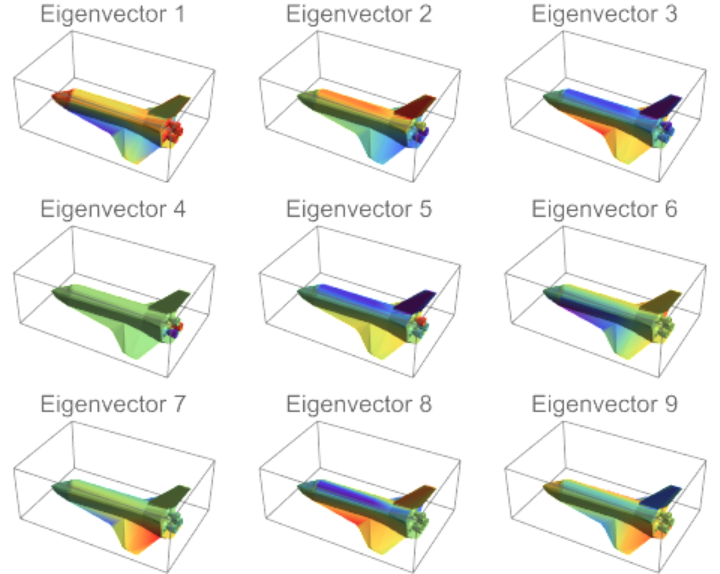### Applications (1)

The Laplace-Beltrami operator can be used to compute how the heat is diffused on a surface. In this example, the heat kernel signature (HKS) is computed from a heat impulse:

 In:=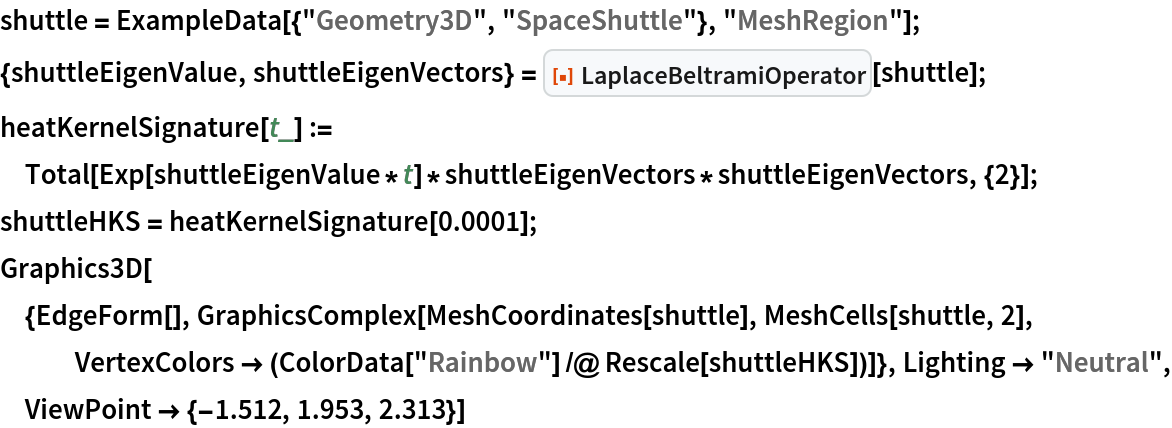Out=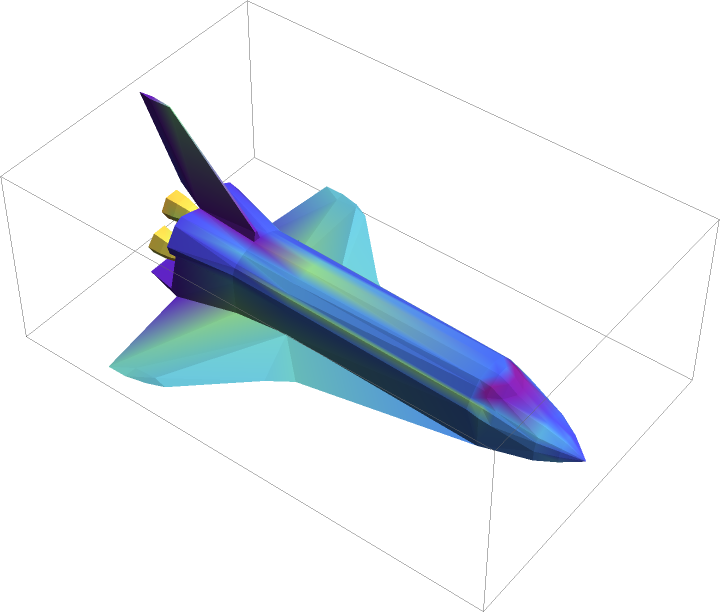Gabriel Lemieux

## Version History

• 1.0.0 – 13 February 2023